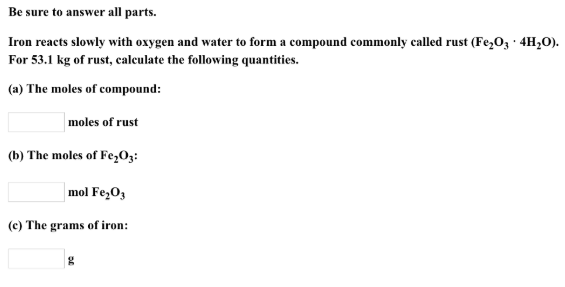# Problem: Be sure to answer all parts.Iron reacts slowly with oxygen and water to form a compound commonly called rust (Fe2O3•4H3O). For 53.1 kg of rust, calculate the following quantities (a) The moles of compound: (b) The moles of Fe2O3: (c) The grams of iron:

###### FREE Expert Solution
84% (121 ratings)###### Problem Details

Be sure to answer all parts.

Iron reacts slowly with oxygen and water to form a compound commonly called rust (Fe2O3•4H3O).

For 53.1 kg of rust, calculate the following quantities

(a) The moles of compound:

(b) The moles of Fe2O3

(c) The grams of iron: